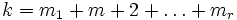# Classification of finite abelian groups

(diff) ← Older revision | Latest revision (diff) | Newer revision → (diff)
This is a survey article related to:Abelianness
View other survey articles about Abelianness

## Goal

• Describing each finite Abelian group in an easy way from which all questions about its structure can be answered
• For every natural number, giving a complete list of all the isomorphism classes of Abelian groups having that natural number as order.

## Structure theorem

Further information: structure theorem for finitely generated Abelian groups

This theorem is the main result that gives the complete classification. We state it here in a form that is suited for the classification:

Every finite Abelian group can be expressed as a product of cyclic groups of prime power order. Moreover this expression is unique upto ordering of the factors and upto isomorphism

## Classification

### Reduction to case of prime power order groups

The above theorem also tells us that a finite Abelian group is expressible as a direct product of its Sylow subgroups, so it suffices for us to classify all Abelian groups of prime power order.

### Dependence on partitions of the exponent

If an Abelian group of prime power order$p^k$ is expressed as a direct product of cyclic groups of prime power order then the sum of the exponents of all the direct factors equals$k$. Conversely, given any partition of$k$ into nonnegative integers, say$k = m_1 + m+2 + \ldots + m_r$, we can form an Abelian group:$\Z/m_1\Z \times \Z/m_2\Z \times \ldots \Z/m_r\Z$

Thus the set of Abelian groups of order$p^k$ is in bijection with the set of nonnegative integer partitions of$k$.

### The overall description

Let$n = p_1^{k_1}p_2^{k_2} \ldots p_t^{k_t}$

Then the set of Abelian groups of order$n$ is in bijective correspondence with$P(k_1) \times P(k_2) \times \ldots \times P(k_t)$ where$P(m)$ denotes the set of unordered partitions of the integer$m$ into nonnegative integer parts.